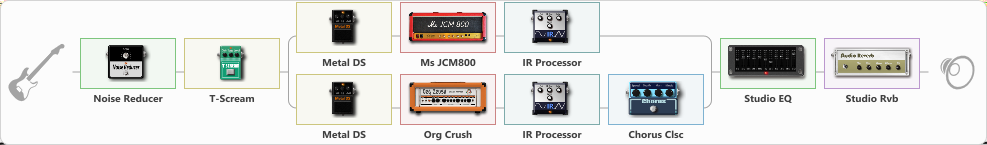# Metallica Ride The Lightning tone

Discussion in 'ToneLib-GFX presets' started by Tony the Metalhead, Oct 30, 2021.

1. ### Tony the MetalheadActive Member

Metallica Ride The Lightning tone

Preset name: I Have Lost The Will To Live

Updated
Try "For whom the bell tollz"

Effects chain:Effect: "Noise Reducer" (Dynamics / Filter), active - "yes"
{
"Sens" = 65
"Mode" = Hard
}

Effect: "T-Scream" (Overdrive / Distortion), active - "yes"
{
"Drive" = 55
"Tone" = 50
"Level" = 20
}

Effect: "Splitter" (Dynamics / Filter)
{
"A-Bypass" = Off
"A-Pan" = 0
"A-Level" = 55
"B-Bypass" = Off
"B-Pan" = 0
"B-Level" = 55

'A' branch:
{

Effect: "Metal DS" (Overdrive / Distortion), active - "yes"
{
"Dist" = 70
"Bass" = 50
"Middle" = 55
"Treble" = 65
"Level" = 25
}

Effect: "Ms JCM800" (Amp simulators), active - "yes"
{
"Gain" = 80
"Bass" = 50
"Middle" = 60
"Treble" = 40
"Presence" = 55
"Master" = 35
"Level (dB)" = -3
}

Effect: "IR Processor" (Cabinets), active - "yes"
{
"IR" = ride_IR
"Low Cut (Hz)" = 125
"Hi Cut (kHz)" = 6.5
"Mix" = 100
"Level (dB)" = 3
}
}
'B' branch:
{

Effect: "Metal DS" (Overdrive / Distortion), active - "yes"
{
"Dist" = 75
"Bass" = 55
"Middle" = 45
"Treble" = 65
"Level" = 20
}

Effect: "Org Crush" (Amp simulators), active - "yes"
{
"Gain" = 75
"Bass" = 45
"Middle" = 60
"Treble" = 40
"Presence" = 55
"Master" = 35
"Level (dB)" = -3
}

Effect: "IR Processor" (Cabinets), active - "yes"
{
"IR" = ride_IR
"Low Cut (Hz)" = 175
"Hi Cut (kHz)" = 6.5
"Mix" = 100
"Level (dB)" = 0
}

Effect: "Chorus Clsc" (Modulation / Sfx), active - "yes"
{
"Speed" = 1.5
"Depth" = 25
"Center" = 2.5
"Mode" = Stereo
}
}
}

Effect: "Studio EQ" (Dynamics / Filter), active - "yes"
{
"31 Hz" = -12
"62 Hz" = -4
"125 Hz" = 2
"250 Hz" = 1
"500 Hz" = -3
"1 kHz" = -5
"2 kHz" = 5
"4 kHz" = 6
"8 kHz" = 3
"16 kHz" = -8
"Level (dB)" = 1
}

Effect: "Studio Rvb" (Reverberation), active - "yes"
{
"Time" = 2.0
"PreDelay" = 5
"LoDamp" = 5
"HiDamp" = 10
"Mix" = 15
}

Note: You will need to download and install the ToneLib-GFX software to use the preset.

File size:
2 KB
Views:
2,150